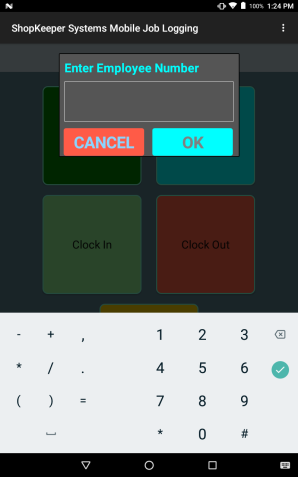# Android QuestionResize [B4X] Input dialogs with XUI Views

#### MrKim

##### Well-Known Member
Spent quite a bit of time (with help from several people here - thank you all) figuring out how to resize an input dialog and get just the input I want. It is not exactly what I want yet but it is a good start.Thought I would post what I have done in case others find it useful.

B4X:
``````Sub YourSub

Dim input As B4XInputTemplate
input.Initialize
input.RegexPattern = ".+" 'require at least one character
input.lblTitle.Text = "Enter Employee Number"
input.mBase.Height = 20%y
input.mBase.Width = 60%x
Dim RS As ResumableSub = (dialog.ShowTemplate(input, "OK", "", "CANCEL"))
FormatDialog(input, True, False)
Wait For(RS) Complete (Result As Int)
If Result = xui.DialogResponse_Positive Then
.
.
Else
.
.
End If
End Sub
.
.
.
Sub  FormatDialog(input As B4XInputTemplate, NumOnly As Boolean, PW As Boolean)
Dim ET As EditText = input.TextField1
Dim p As Panel = input.GetPanel(dialog)
ET.Height = (input.mBase.Height / 2)
ET.top = (input.mBase.Height / 3)
ET.Width = input.mBase.Width - (ET.Left * 2)
Dim p As Panel = input.GetPanel(dialog)
Dim LB As Label = p.GetView(0)
LB.Height = (input.mBase.Height / 3)
LB.TextSize = 25
LB.TextColor = Colors.Cyan
Dim Cncl As B4XView = dialog.GetButton(xui.DialogResponse_Cancel)
Cncl.Width = (input.mBase.Width * .45)
Cncl.Left = ET.Left
Cncl.Height = Cncl.Height * 1.2  '((p.Height - (ET.Top + ET.Height)) * 2) '- 5dip        '
Cncl.TextSize = 33
Cncl.Color = Colors.RGB(255, 91, 71)
Cncl.Top = ET.Top + ET.Height + 15dip
Dim ok As B4XView = dialog.GetButton(xui.DialogResponse_Positive)
ok.Width = Cncl.Width
ok.Left = ET.Left + ET.Width - ok.Width
ok.Height = Cncl.Height
ok.TextSize = 33
ok.Color = Colors.Cyan
ok.Top = ET.Top + ET.Height + 15dip
ET.TextSize = 32
If NumOnly Then
'input.ConfigureForNumbers(True, False)
IME.SetCustomFilter(ET, ET.INPUT_TYPE_NUMBERS, "-.0123456789")
Else
ET.InputType = Bit.Or(128, Bit.Or(ET.InputType, 524288)) 'VARIATION_PASSWORD, NO_SUGGESTION
End If
End Sub``````
Biggie for me:
B4X:
``````Dim RS As ResumableSub = (dialog.ShowTemplate(input, "OK", "", "CANCEL"))
.
.
Wait For(RS) Complete (Result As Int)``````
WOW! How to open a "Modal" Dialog and alter it after it is open! Never would have occurred to me you could do that.

Gotcha:
B4X:
``````input.mBase.Height = 20%y
input.mBase.Width = 60%x``````
Have to set these before you open the dialog. Sets the overall size.

Last edited:
•Erel and jimmyF

#### Erel

Staff member
Thank you for sharing this. It is worth adding a screenshot and posting it under the code snippets forum.

•MrKim

#### MrKim

##### Well-Known Member
Thank you for sharing this. It is worth adding a screenshot and posting it under the code snippets forum.
Thanks Erel, the one part I can't get is scaling the button height. It seems like (pseudocode):
B4X:
``input.mBase.Height - (input.TextField1.Top + input.TextField1.Height)``
Should give me the space between the bottom of TextField1 and the bottom of the dialog but it doesn't.

Also, (just curious) what is the difference between input.mBase and input.GetPanel(dialog)

Thanks

#### Erel

•